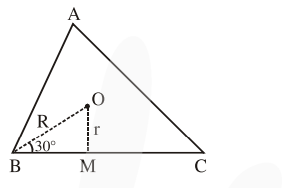# Let the centroid of an equilateral triangle

Question:

Let the centroid of an equilateral triangle $\mathrm{ABC}$ be at the origin. Let one of the sides of the equilateral triangle be along the straight line $x+y=3 .$ If $R$ and $r$ be the radius of circumcircle and incircle respectively of $\triangle \mathrm{ABC}$, then $(\mathrm{R}+\mathrm{r})$ is equal to :

1. $\frac{9}{\sqrt{2}}$

2. $7 \sqrt{2}$

3. $2 \sqrt{2}$

4. $3 \sqrt{2}$

Correct Option: 1

Solution:$\mathrm{r}=\mathrm{OM}=\frac{3}{\sqrt{2}}$

$\& \sin 30^{\circ}=\frac{1}{2}=\frac{\mathrm{r}}{\mathrm{R}} \Rightarrow \mathrm{R}=\frac{6}{\sqrt{2}}$

$\therefore r+R=\frac{9}{\sqrt{2}}$